# PSAT Math : How to find a fraction from a percentage

## Example Questions

### Example Question #3 : Fractions And Percentage

Find the simplified fraction for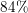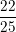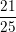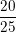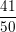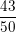Explanation:

For any percent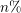we can write it as a fraction in the form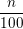So we can writeas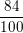Then we simplify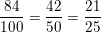So our simplified answer is